# Climate Mathematics: Theory and Applications¶

Samuel S.P. Shen ad Richard C.J. Somerville

This Version 2.0 is authored by Briana Ramirez, edited by Samuel Shen. Liu Yang and Sandra Villamar contributed codes to this version.

Video tutorial for the python code can be found at the following URL:

This version is based upon the previous version described in the following box.

In :
######################################################################################################################
#This Python Code for Climate Science is written for the book entitled "Climate Mathematics: Theory and Applications"#
#A Cambridge University Press book authored by SSP Shen and RCJ Somerville in July 2019                              #
#The Python codes were based on the R codes written by Samuel Shen Distinguished Professor,                          #
#San Diego State University, USA and were translated from R by Louis Selstad, Stephen Shen,                          #
#Gregori Clarke, and Dakota Newmann and edited by Samuel Shen.                                                       #
######################################################################################################################

In :
#FIRST TIME Python users*****
#These package need to be installed (on the terminal or anaconda interface) before importing them below.

#Follow this tutorial for package installation before
# https://towardsdatascience.com/importerror-no-module-named-xyz-45e4a5339e1b

In :
#Change your file path to the folder where your downloaded data is stored
#MAC HELP: https://support.apple.com/guide/mac-help/go-directly-to-a-specific-folder-on-mac-mchlp1236/mac
#PC HELP: https://www.sony.com/electronics/support/articles/00015251
import os

In :
%%javascript
IPython.OutputArea.prototype._should_scroll = function(lines) {
return false;
}

In :
#Style Dictionary to standarize plotting scheme between different python scripts
import matplotlib.pyplot as plt

styledict = {'xtick.labelsize':20,
'xtick.major.size':9,
'xtick.major.width':1,
'ytick.labelsize':20,
'ytick.major.size':9,
'ytick.major.width':1,
'legend.framealpha':0.0,
'legend.fontsize':15,
'axes.labelsize':20,
'axes.titlesize':25,
'axes.linewidth':2,
'figure.figsize':(12,8),
'savefig.format':'jpg'}
plt.rcParams.update(**styledict)

In :
#Function that creates personalized discrete Colormap
import numpy as np
from matplotlib import cm as cm1
from matplotlib.colors import ListedColormap, to_rgba

def newColMap(colors):
"""
This function creates a new color map from a list of colors given
as a parameter. Recommended length of list of colors is at least 6.
"""
first = np.repeat([to_rgba(colors)], 2, axis = 0)
last = np.repeat([to_rgba(colors[-1])], 2, axis = 0)
v = cm1.get_cmap('viridis', 16*(len(colors)-2))
newcolors = v(np.linspace(0, 1, 16*(len(colors)-2)))
for (i, col) in enumerate(colors[1:-1]):
newcolors[16*i : 16*(i+1), :] = to_rgba(col)
return ListedColormap(np.append(np.append(first,newcolors, axis=0), last, axis=0))


# Chapter 2: Basics of Python Programming¶

In :
import numpy as np
import matplotlib.pyplot as plt
from matplotlib.colors import rgb2hex
from matplotlib.patches import Polygon
import matplotlib.mlab as mlab
import pandas as pd
from mpl_toolkits.basemap import Basemap
# Having an issue with the import “mpl_toolkits.basemap” package?
# Unlike other package installations, this package requires additional steps to install.
#      https://www.lfd.uci.edu/~gohlke/pythonlibs/#basemap
#          *Note the package version needs to be the same as Python version
#    2.Run the following line in your terminal
#      pip install basemap-1.2.2-cp37-cp37m-win_amd64.whl

from mpl_toolkits import mplot3d
from netCDF4 import Dataset as ds
from urllib import request
import scipy as sp
from scipy import stats as stt
from sklearn import datasets, linear_model
from sklearn.neighbors import KernelDensity as kd
from sklearn.linear_model import LinearRegression
from random import randint
from scipy.stats import norm
import statsmodels
import sklearn
import math
import statistics
import sympy as sy
from sympy import symbols, diff
import statsmodels.api as sm
from datetime import date


# 2.2: Python Tutorial¶

Python As a Smart Calculator

In :
# Basic arithmetic
1+4

Out:
5
In :
# Basic arithmetic
2 + np.pi/4 - 0.8

Out:
1.9853981633974482
In :
# Variable Assignment
x = -1
y = 2
z = 4

# x**y means x^y: x to the power of y
t = 2*x**y-z
t

Out:
-2
In :
# Variable Assignment
u=2
v=3
u+v

Out:
5
In :
# np.sin is sine function in Python
np.sin(u*v)

Out:
-0.27941549819892586

Define a Sequence in Python

In :
# Enter the temperature data
tmax=[77, 72,75,73,66,64,59]

# Show the temperarure data
tmax

Out:
[77, 72, 75, 73, 66, 64, 59]
In :
# A Python sequence starts from 0 while R from 1
np.arange(9)
# A sequence is called an array in Python

Out:
array([0, 1, 2, 3, 4, 5, 6, 7, 8])
In :
# np.arange(x,y) creates an array of numbers incremented by 1 between integer x and integer y-1
np.arange(1, 9)

Out:
array([1, 2, 3, 4, 5, 6, 7, 8])
In :
# np.arange(x,y) can take negative and real numbers with increment 1
np.arange(-5.5, 2)

Out:
array([-5.5, -4.5, -3.5, -2.5, -1.5, -0.5,  0.5,  1.5])

Define a Function in Python

In :
# Define the x^2 function and call it samfctn(x)
def samfctn(x):
return x * x
samfctn(4)

Out:
16
In :
#Fig 2.3
x = np.linspace(1,8,7)
tmax=[77, 72,75,73,66,64,59]

plt.plot(x, tmax, "o")

Out:
[<matplotlib.lines.Line2D at 0x7fe0f713aa90>]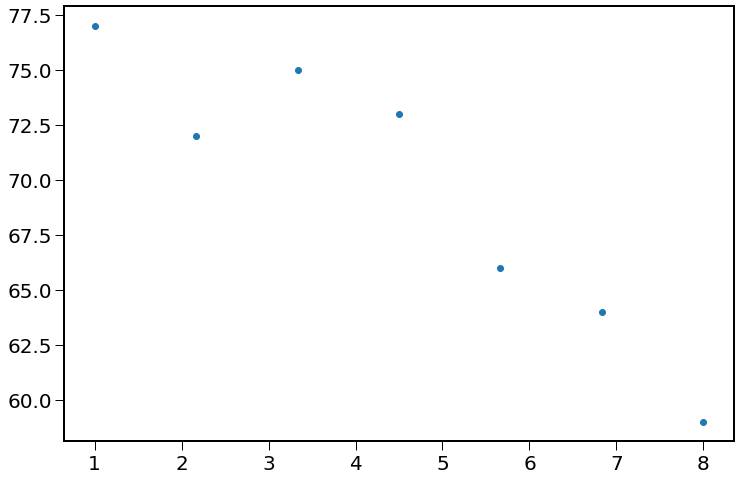In :
# Define a multivaraite function "x+y-z/2"
def fctn2(x, y, z):
return x + y - z / 2;
fctn2(1, 2, 3)

Out:
1.5

Plot with Python

Plot the curve of y=sin(x) from -pi to 2 pi

In :
# math.pi is the same as np.pi and scipy.pi, just using different packages
t = np.arange(-math.pi, 2 *math.pi, 0.1)
plt.plot(t, np.sin(t),"o")
plt.show()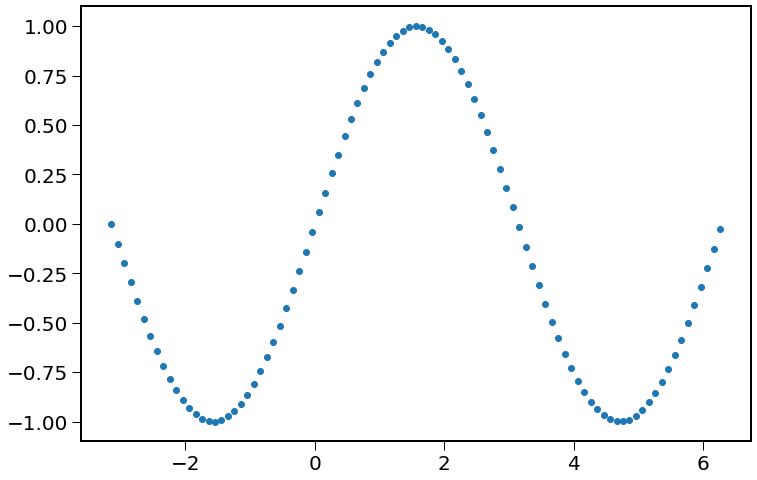Plot x-y data based on a formula

In :
# Uniform division using 20 points
t = np.linspace(-3, 2, 20)
plt.plot(t, t ** 2)
plt.show()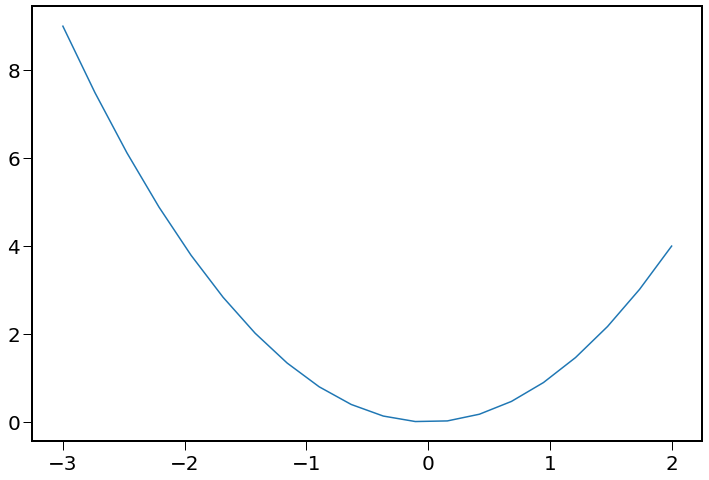Plot 3D Surface

In :
x = np.linspace(-1, 1, 100)
y = np.linspace(-1, 1, 100)

# Defines a formula for Z
def f(x, y):
return 1 - x**2 - y**2

X, Y = np.meshgrid(x, y)

# Renders z function on the x, y grid
Z = f(X, Y)

# Creates the 3D plot
fig = plt.figure()
ax = plt.axes(projection = '3d')
ax.contour3D(X, Y, Z, 30, cmap = 'binary')
plt.show()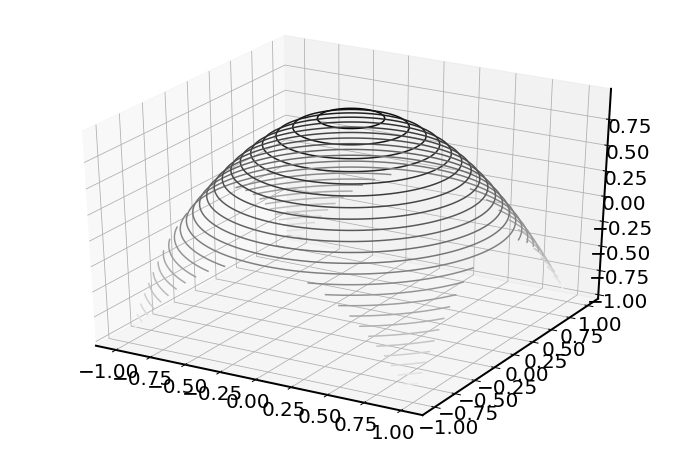Contour plot

In :
#Fig 2.4
x = np.linspace(-1, 1, 30)
y = np.linspace(-1, 1, 30)

X, Y = np.meshgrid(x, y)
Z = f(X, Y)

# Creates and colors the contour plot
plt.contourf(X, Y, Z, 20, cmap = 'cool') #cmap='color-scheme'

# Adds colorbar for color map of contours
plt.colorbar()
plt.show()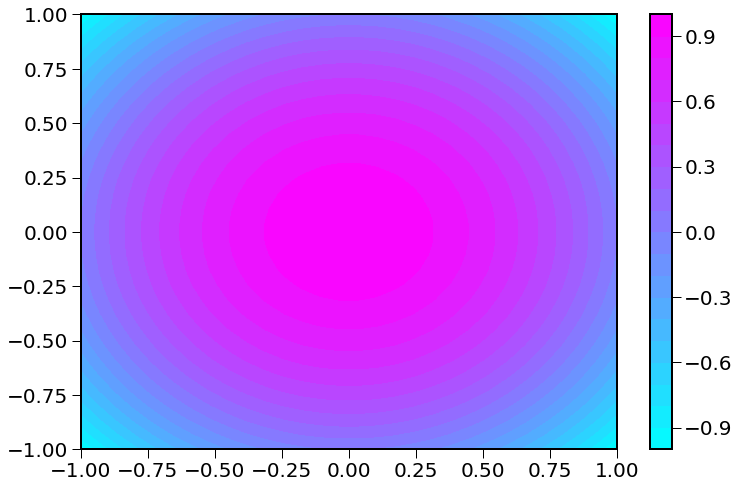Plot 3D Contour Plot

In :
x = np.linspace(-1, 1, 100)
y = np.linspace(-1, 1, 100)

# Defines a formula for Z
def f(x, y):
return 1 - x**2 - y**2

X, Y = np.meshgrid(x, y)

# Renders z function on the x, y grid
Z = f(X, Y)

# Creates the 3D plot
fig = plt.figure()
ax = plt.axes(projection = '3d')
ax.plot_surface(X, Y, Z, rstride=1, cstride=1,
cmap='viridis', edgecolor='none') #cmap = 'color'

# Labels the plot
ax.set_title('Contour surface')
plt.xticks(fontsize=14)
plt.yticks(fontsize=14)
ax.set_zticklabels([-1,-.75,-.5,-.25,0,.25,.5,.75,1],Fontsize=14)
ax.view_init(30)
plt.show()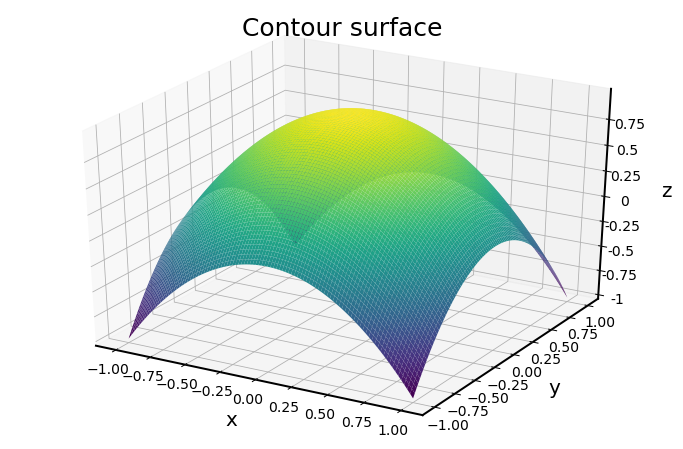Symbolic Calculations with Python

In :
# Makes 'x' and 'y' symbols used for derivatives
x = symbols('x')
y = symbols('y')
# 'diff' command takes the derivative of x^2 w.r.t. x
diff(x ** 2, x)

Out:
$\displaystyle 2 x$
In :
# Creates a function of x^2
fx = x ** 2

# Takes the derivative of function w.r.t x
rslt = diff(fx, x)
print('The derivative of the function fx w.r.t x is: ', rslt)

The derivative of the function fx w.r.t x is:  2*x

In :
# Creates a function of x^2*sin(x)
fx = (x ** 2) * sy.sin(x)

# Take the derivative of function w.r.t x
rslt = diff(fx, x)
print('The derivative of the function w.r.t x is: ', rslt)

The derivative of the function w.r.t x is:  x**2*cos(x) + 2*x*sin(x)

In :
# Create a multivariate function: x^2+y^2
fxy = x ** 2 + y ** 2

# Take partial derivative of function w.r.t x
rslt_x = diff(fxy, x)

# Take partial derivative of function w.r.t y
rslt_y = diff(fxy, y)
print('The partial derivative of the function w.r.t x is: ', rslt_x)
print('The partial derivative of the function w.r.t y is: ', rslt_y)

The partial derivative of the function w.r.t x is:  2*x
The partial derivative of the function w.r.t y is:  2*y

In :
# Integrates x^2 from 0 to 1
sy.integrate(x ** 2, (x, 0, 1))

Out:
$\displaystyle \frac{1}{3}$
In :
# Integrates cos(x) from 0 to pi/2
sy.integrate(sy.cos(x), (x, 0, math.pi / 2))

Out:
$\displaystyle 1.0$

Vectors and Matrices

In :
# Creates a 5X1 matrix
np.mat([, , , [np.pi], [-3]])

Out:
matrix([[ 1.        ],
[ 6.        ],
[ 3.        ],
[ 3.14159265],
[-3.        ]])
In :
# Creates a sequence from 2 to 6
np.arange(2, 7)

Out:
array([2, 3, 4, 5, 6])
In :
# Creates a sequence incremented by 2 from 1 to 10
np.arange(1, 10, 2)

Out:
array([1, 3, 5, 7, 9])
In :
# Creates a 4X1 matrix
x = np.mat([, [-1], , [-1]])

# Adds number 1 to each element of x
print(x + 1)

[


]

In :
# Multiplies number 2 to each element of x
print(x * 2)

[[ 2]
[-2]
[ 2]
[-2]]

In :
# Divides each element of x by 2
print(x / 2)

[[ 0.5]
[-0.5]
[ 0.5]
[-0.5]]

In :
# Creates a 1X4 vector with a sequence incremented by 1 from 1 to 4
y = np.mat(np.arange(1, 5))

# This multiplication multiples each pair of elements and results in 4X1 vector
np.multiply(x, y.T)

Out:
matrix([[ 1],
[-2],
[ 3],
[-4]])
In :
# Dot product and results in 1X1 matrix
np.dot(x.T, y.T)

Out:
matrix([[-2]])
In :
# Transforms x into a row 1X4 vector
x.T.shape

Out:
(1, 4)
In :
#The dot product of two vectors
np.dot(y, x)

Out:
matrix([[-2]])
In :
#This column times row yields a 4X4 matrix
# Note: For detailed difference between np.multiply and np.dot visit the following URL:
#    https://www.geeksforgeeks.org/difference-between-numpy-dot-and-operation-in-python/

np.matmul(x, y)

Out:
matrix([[ 1,  2,  3,  4],
[-1, -2, -3, -4],
[ 1,  2,  3,  4],
[-1, -2, -3, -4]])
In :
# Convert a vector into a matrix of the same number of elements
# the matrix elements go by column, first column, second, etc
my = y.reshape(2, 2).transpose()
print(my)

[[1 3]
[2 4]]

In :
# Finds dimensions of a matrix
my.shape

Out:
(2, 2)
In :
# Converts a matrix to a vector, again via columns
my.flatten()

Out:
matrix([[1, 3, 2, 4]])
In :
# Reshapes x to a 2X2 matrix
mx = x.reshape(2, -1).transpose()
# Multiplication between each pair of elements
np.multiply(mx, my)

Out:
matrix([[ 1,  3],
[-2, -4]])
In :
# Division between each pair of elements
np.divide(mx, my)

Out:
matrix([[ 1.        ,  0.33333333],
[-0.5       , -0.25      ]])
In :
# Subtract 2 times a matrix from another
np.subtract(mx, 2 * my)

Out:
matrix([[-1, -5],
[-5, -9]])
In :
# This is matrix multiplication in linear algebra
np.matmul(mx, my)

Out:
matrix([[ 3,  7],
[-3, -7]])
In :
# Determinant of a matrix
x = np.linalg.det(my)
print(x)

-2.0

In :
# Inverse of a matrix
z = np.linalg.inv(my)
print(z)

[[-2.   1.5]
[ 1.  -0.5]]

In :
#Verifies that the inverse of the matrix is correct by multiplying
# the original matrix and the inverse matrix
# Note: np.multiply is element-wise multiplication and np.matmul is matrix multiplication
a = np.matmul(z, my)
print(a)

[[1. 0.]
[0. 1.]]

In :
# Returns a left to right diagonal vector of a matrix
np.diagonal(my)

Out:
array([1, 4])
In :
# Returns the eigenvalues and eigenvectors of the "my" matrix
myeigvect = np.linalg.eig(my)
print(myeigvect)

(array([-0.37228132,  5.37228132]), matrix([[-0.90937671, -0.56576746],
[ 0.41597356, -0.82456484]]))

In :
# Returns the singular value decomposition(svd) of the "my" matrix
mysvd = np.linalg.svd(my)
print(mysvd)

(matrix([[-0.57604844, -0.81741556],
[-0.81741556,  0.57604844]]), array([5.4649857 , 0.36596619]), matrix([[-0.40455358, -0.9145143 ],
[ 0.9145143 , -0.40455358]]))

In :
# Returns a solved linear matrix equation of the "my" matrix and array [1,3]
ysolve = np.linalg.solve(my, [1,3])
print(ysolve)

[ 2.5 -0.5]

In :
# Verifies the solution is correct by multiplying the "my" matrix with "ysolve"
n = np.matmul(my, ysolve)
print(n)

[[1. 3.]]


Simple Statistics with Python

In :
# Generates 10 normally distributed numbers
random = np.random.normal(0, 1, 10)
print(random)

[ 0.11772201  0.42658008  1.38953522  0.10200311  1.50857981 -2.05713206
1.17927559 -0.29979936  1.68308386  2.99186445]

In :
# Returns the mean of that random sample
mean = np.mean(random)
print(mean)

0.7041712701352237

In :
# Returns the variance of that random sample
var = np.var(random)
print(var)

1.6950670069236646

In :
# Returns the standard deviation of that random sample
std = np.std(random)
print(std)

1.3019473902288312

In :
# returns the median of that random sample
median = np.median(random)
print(median)

0.8029278339939724

In :
#Returns quantile values in [0,.25,.5,.75,1]
quantile = pd.DataFrame(random).quantile([0, 0.25, 0.5, 0.75, 1])
print(quantile)

             0
0.00 -2.057132
0.25  0.105933
0.50  0.802928
0.75  1.478819
1.00  2.991864

In :
# Returns range of values (max - min) in random sample
rang = np.ptp(random)
print(rang)

5.048996504102041

In :
# Returns the min value in random
minimum = np.min(random)
print(minimum)

# Returns the max value in random
maximum = np.max(random)
print(maximum)

-2.057132056343103
2.9918644477589376

In :
# Yields the box plot of random values
fig = plt.figure(1, figsize=(8, 10))
bp = ax.boxplot(random)
plt.show()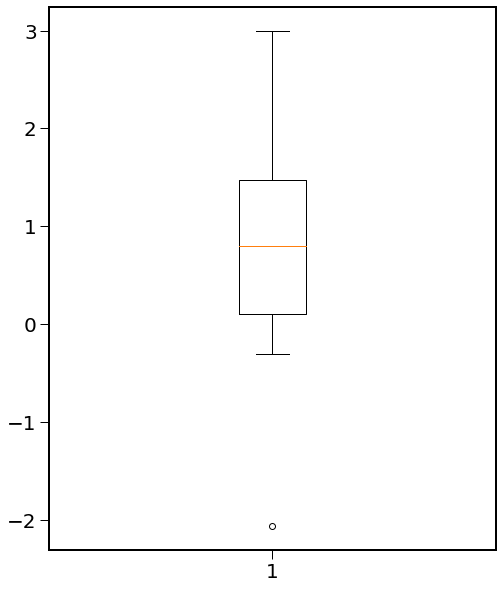In :
# Yeilds statistical summary of the data sequence
random = np.random.normal(0, 1, 12)
summ = pd.DataFrame(random).describe()
print(summ)

               0
count  12.000000
mean   -0.433629
std     0.836414
min    -1.828387
25%    -1.104349
50%    -0.371238
75%     0.079492
max     0.872097

In :
# Yields the histogram of 1000 random numbers with a normal distribution
w = np.random.normal(0, 1, 1000)
hist = plt.hist(w, facecolor='black')
plt.show()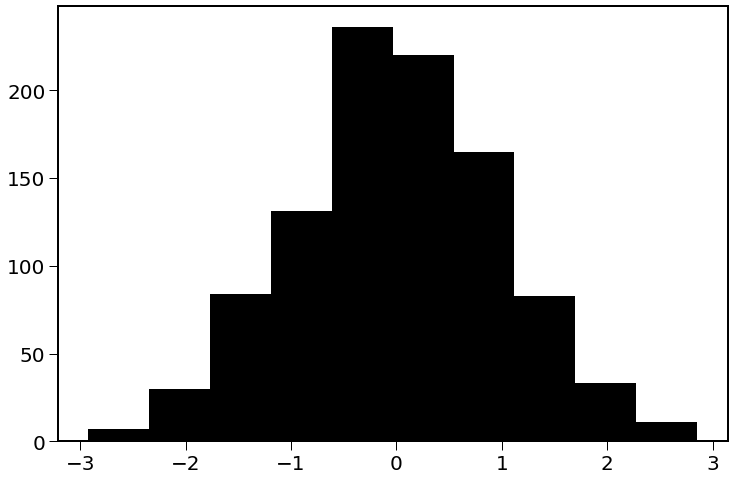In :
#Fig 2.5
# Linear Regression and linear trend line
# 2007-2016 data of global temperature anamolies
# Source: NOAAGlobalTemp data
from sklearn.linear_model import LinearRegression

t = np.linspace(2007, 2016, 10)
T = [.36,.3,.39,.46,.33,.38,.42,.5,.66,.7]
lm = LinearRegression()
lm.fit(t.reshape(-1,1), T)
predictions = lm.predict(t.reshape(-1, 1))

plt.plot(t, predictions, '-r')
plt.plot(t,T,"-o", color="black")
plt.title("2007-2016 Global Temperature Anomalies")
plt.xlabel("Year")
plt.ylabel("Temperature")
plt.show()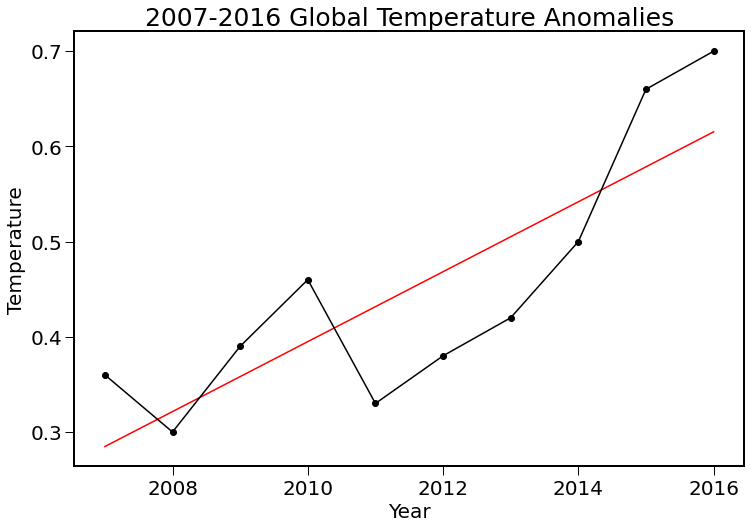Input Data by reading a file

In :
# CSV file
file = "NOAAGlobalT.csv"

In :
# TXT file
file = 'http://shen.sdsu.edu/data/' + 'aravg.mon.land_ocean.90S.90N.v4.0.1.201703.asc.txt'

In :
# ASC file
file = open('NOAAGlobalTemp.gridded.v4.0.1.201701.asc', "r")
# read float numbers from the file
data = []
for line in file:
x = line.strip().split()
for f in x:
data.append(float(f))

In :
# NetCDF file
file = "air.mon.mean.nc"
data = ds(file,"r+")

In [ ]:



# Chapter 3: Basic Statistical Methods for Climate Data Analysis¶

In :
import numpy as np
import matplotlib.pyplot as plt
from matplotlib.colors import rgb2hex
from matplotlib.patches import Polygon
import matplotlib.mlab as mlab
import pandas as pd
from mpl_toolkits.basemap import Basemap
from mpl_toolkits import mplot3d
from netCDF4 import Dataset as ds
from urllib import request
import scipy as sp
from scipy import stats as stt
from sklearn import datasets, linear_model
from sklearn.neighbors import KernelDensity as kd
from sklearn.linear_model import LinearRegression
from random import randint
from scipy.stats import norm
import statsmodels
import sklearn
import math
import statistics
import sympy as sy
from sympy import symbols, diff
import statsmodels.api as sm
from datetime import date
from scipy.stats import norm, kurtosis
from sklearn.linear_model import LinearRegression
import seaborn as sns
import statsmodels.api as sm
import pylab as py
from scipy.stats import norm, t
import warnings
warnings.filterwarnings("ignore")


# 3.1: Statistical Indices from Global Temperature Data from 1880 to 2015¶

In :
# download the NCEI spatial average time series of monthly data
# https://www1.ncdc.noaa.gov/pub/data/noaaglobaltemp/operational/
# timeseries/aravg.mon.land_ocean.90S.90N.v4.0.1.201702.asc
'aravg.mon.land_ocean.90S.90N.v4.0.1.201703.asc.txt',
header = None, delim_whitespace = True)
aveNCEI = aveNCEI.values
aveNCEI

Out:
array([[ 1.88000e+03,  1.00000e+00, -2.54882e-01, ...,  1.25049e-01,
1.18830e-01,  1.50000e-02],
[ 1.88000e+03,  2.00000e+00, -3.90030e-01, ...,  1.69170e-01,
8.25840e-02,  8.65860e-02],
[ 1.88000e+03,  3.00000e+00, -4.14509e-01, ...,  1.28022e-01,
8.60760e-02,  4.19460e-02],
...,
[ 2.01700e+03,  1.00000e+00,  6.36712e-01, ...,  1.25049e-01,
1.28754e-01,  1.50000e-02],
[ 2.01700e+03,  2.00000e+00,  6.96222e-01, ...,  1.69170e-01,
1.81365e-01,  1.50000e-02],
[ 2.01700e+03,  3.00000e+00,  7.69576e-01, ...,  1.28022e-01,
1.61637e-01,  3.36150e-02]])

# Mean, Variance, Standard Deviation, Skewness, Kurtosis, and Quantiles¶

In :
# Yields the dimensions of data
aveNCEI.shape

Out:
(1647, 10)
In :
# Take only the second column of this data matrix
tmean15 = aveNCEI[:,2]

# Yields the first 6 values
tmean15[:6]

Out:
array([-0.254882, -0.39003 , -0.414509, -0.314104, -0.327618, -0.421961])
In :
# Yields the sample Mean
np.mean(tmean15)

Out:
-0.19504468609593198
In :
# Yields the sample Standard deviation
np.std(tmean15)

Out:
0.3289373979136597
In :
# Yields the sample Variance
np.var(tmean15)

Out:
0.10819981174620931
In :
# Yields the sample Skewness
pd.DataFrame(tmean15).skew()

Out:
0    0.657679
dtype: float64
In :
# Yields the sample Kurtosis
kurtosis(tmean15)

Out:
-0.09874723968157051
In :
# Yields the sample Median
np.median(tmean15)

Out:
-0.263387
In :
# Yields desired quantiles
pd.DataFrame(tmean15).quantile([0, 0.25, 0.5, 0.75, 1])

Out:
0
0.00 -0.934475
0.25 -0.440763
0.50 -0.263387
0.75 0.006320
1.00 0.949248

# Correlation, Covariance, and Linear Trend¶

In :
# Creating a year sequence
timemo = np.linspace(1880, 2015, 1647)

# Creating Liner Regression
lm = LinearRegression()

# Training the model
lm.fit(timemo.reshape(-1, 1), tmean15)

# Using the model to make predictions
predictions = lm.predict(timemo.reshape(-1, 1))

print("Coeff of Linear Model:", lm.coef_)
print("Intercept of Linear Model: ", lm.intercept_)

Coeff of Linear Model: [0.00698074]
Intercept of Linear Model:  -13.790040882746423

In :
#Fig 3.1
# Linear Model Visualization
plt.close()

plt.plot(timemo, tmean15, '-', color="black")
plt.title('Global Annual Mean Land and Ocean Surface \n Temperature Anomalies: 1880-2015')
plt.xlabel('Year')
plt.ylabel('Temperature anomaly [$\degree$C]')

plt.plot(timemo, predictions, '-', color="red")
font = {'family': 'serif',
'color':  'red',
'weight': 'normal',
'size': 18,
}
plt.text(1885, .5, 'Linear trend: 0.69 [$\degree$C] per century', fontdict = font)

plt.show()